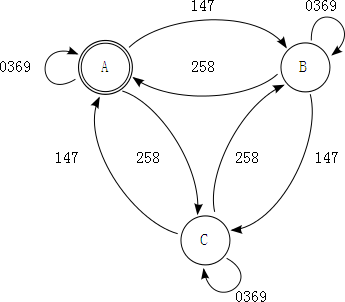# 自学编程难不难系列 之 正则(2)..

```作者: 一了 <1liao3@funlang.org>

^(?:(*+)|((?1))(((?1))|(?2)(?5))|(?4)((?2)|(?4)(?3)))+\$(?2) 回到初始(终止)状态, 或者
(?4) 到 B 状态, 即我们预留的第三组 (?3)```

^(?:
(*+)            (?# 1)
|
((?1))           (?# 2)
(                     (?# 3)
((?1))         (?# 4)
|
(?2)(?5)
)
|
(?4)
(                     (?# 5)
(?2)
|
(?4)(?3)
)
)+\$

var r3 = \$`
^(?:
(*+)            (?# 1)
|
((?1))           (?# 2)
(                     (?# 3)
((?1))         (?# 4)
|
(?2)(?5)
)
|
(?4)
(                     (?# 5)
(?2)
|
(?4)(?3)
)
)+\$
`x;

for i = 1 to 1000 do
var s = '';
var n = 0;
for j = 0.random() to 10000 + 100.random() do
var d = 10.random();
n += d;
s &= d;
end do; #?. s;
if r3.match(s) xor n mod 3 = 0 then
?. s;
end if;
end do; exit;

for i = 0 to 10000 step 1 do
var m = r3.match(i);
if m and i mod 3 <> 0 or not m and i mod 3 = 0 then
?, i;
end if;
end do;```